Test: Semiconductor Physics

# Test: Semiconductor Physics - Electrical Engineering (EE)

Test Description

## 20 Questions MCQ Test RRB JE for Electrical Engineering - Test: Semiconductor Physics

Test: Semiconductor Physics for Electrical Engineering (EE) 2023 is part of RRB JE for Electrical Engineering preparation. The Test: Semiconductor Physics questions and answers have been prepared according to the Electrical Engineering (EE) exam syllabus.The Test: Semiconductor Physics MCQs are made for Electrical Engineering (EE) 2023 Exam. Find important definitions, questions, notes, meanings, examples, exercises, MCQs and online tests for Test: Semiconductor Physics below.
Solutions of Test: Semiconductor Physics questions in English are available as part of our RRB JE for Electrical Engineering for Electrical Engineering (EE) & Test: Semiconductor Physics solutions in Hindi for RRB JE for Electrical Engineering course. Download more important topics, notes, lectures and mock test series for Electrical Engineering (EE) Exam by signing up for free. Attempt Test: Semiconductor Physics | 20 questions in 60 minutes | Mock test for Electrical Engineering (EE) preparation | Free important questions MCQ to study RRB JE for Electrical Engineering for Electrical Engineering (EE) Exam | Download free PDF with solutions
 1 Crore+ students have signed up on EduRev. Have you?
Test: Semiconductor Physics - Question 1

### In germanium semiconductor material at T = 400 K the intrinsic concentration is

Detailed Solution for Test: Semiconductor Physics - Question 1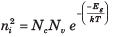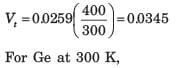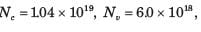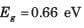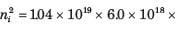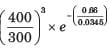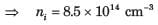Test: Semiconductor Physics - Question 2

### The intrinsic carrier concentration in silicon is to be no greater than ni  = 1x1012 cm-3 . The maximum temperature allowed for the silicon is (Eg = 1.12 eV)

Detailed Solution for Test: Semiconductor Physics - Question 2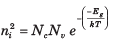(1012)2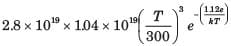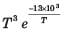= 9.28 x 10-8,  By trial T = 385 K

Test: Semiconductor Physics - Question 3

### Two semiconductor material have exactly the same properties except that material A has a bandgap of 1.0eV and material B has a bandgap energy of 1.2 eV. The ratio of intrinsic concentration of material A to that of material B is

Detailed Solution for Test: Semiconductor Physics - Question 3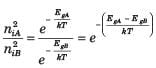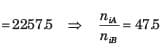Test: Semiconductor Physics - Question 4

In silicon at T = 300 K the thermal-equilibrium concentration of electron is n0 = 5 x 104 cm-3 The hole concentration is

Detailed Solution for Test: Semiconductor Physics - Question 4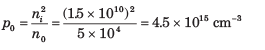Test: Semiconductor Physics - Question 5

In silicon at T = 300 K if the Fermi energy is 0.22 eV above the valence band energy, the value of p0 is

Detailed Solution for Test: Semiconductor Physics - Question 5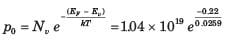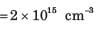Test: Semiconductor Physics - Question 6

The thermal-equilibrium concentration of hole p0 in silicon at T =  300 K is 1015  cm-3. The value of n0 is

Detailed Solution for Test: Semiconductor Physics - Question 6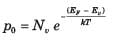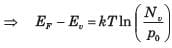At 300 K, Nv = 1.0 x 1019 cm-3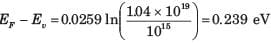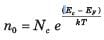At 300 K, Nc = 2.8 x 1019 cm-3
Ec - EF = 1.12 - 0.239 = 0.881 eV
n = 4.4 x 104 cm-3

Test: Semiconductor Physics - Question 7

In germanium semiconductor at T = 300 K, the acceptor concentrations is Na = 1013 cm-3 and donor concentration is Nd = 0. The thermal equilibrium concentration p0 is

Detailed Solution for Test: Semiconductor Physics - Question 7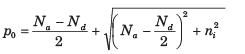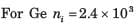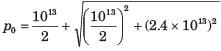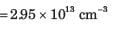Test: Semiconductor Physics - Question 8

A thin film resistor is to be made from a GaAs film doped n - type. The resistor is to have a value of 2 kΩ. The resistor length is to be 200 μm and area is to be 10-6 cm2. The doping efficiency is known to be 90%. The mobility of electrons is 8000 cm2 / V - s . The doping needed is

Detailed Solution for Test: Semiconductor Physics - Question 8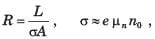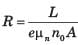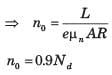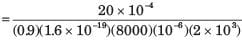Test: Semiconductor Physics - Question 9

A silicon sample doped n - type at 1018 cm-3  have a resistance of 10 Ω . The sample has an area of 10-6 cm2 and a length of 10 μm. The doping efficiency of the sample is (μn =  800 cm2/V - s)

Detailed Solution for Test: Semiconductor Physics - Question 9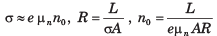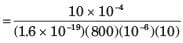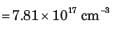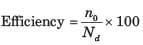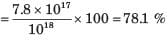Test: Semiconductor Physics - Question 10

Six volts is applied across a 2 cm long semiconductor bar. The average drift velocity is 10cm/s. The electron mobility is

Detailed Solution for Test: Semiconductor Physics - Question 10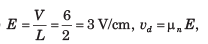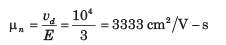Test: Semiconductor Physics - Question 11

For a particular semiconductor material following parameters are observed: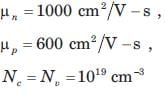These parameters are independent of temperature. The measured conductivity of the intrinsic material is σ = 10-6 (Ω - cm)-1 at T = 300 K. The conductivity at T = 500 K is

Detailed Solution for Test: Semiconductor Physics - Question 11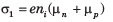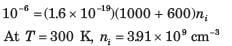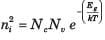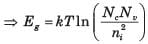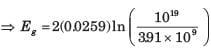= 1.122 eV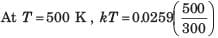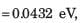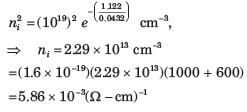Test: Semiconductor Physics - Question 12

An n - type silicon sample has a resistivity of 5 Ω - cm at T = 300 K. The mobility is μn = 1350 cm2 /  V - s. The donor impurity concentration is

Detailed Solution for Test: Semiconductor Physics - Question 12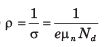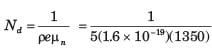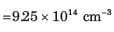Test: Semiconductor Physics - Question 13

In a silicon sample the electron concentration drops linearly from 1018 cm-3  to 1016 cm-3 over a length of 2.0 μm. The current density due to the electron diffusion current is D= 35cm2/s

Detailed Solution for Test: Semiconductor Physics - Question 13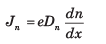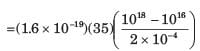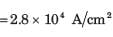Test: Semiconductor Physics - Question 14

In a GaAs sample the electrons are moving under an electric field of 5 kV cm and the carrier concentration is uniform at 1016 cm-3. The electron velocity is the saturated velocity of 107 cm/s. The drift current density is

Detailed Solution for Test: Semiconductor Physics - Question 14

J = evn = (1.6 x 10-19)(107)(1016) = 1.6 x 104 A/cm2

Test: Semiconductor Physics - Question 15

For a sample of GaAs scattering time is τsc =  10-13s and electron’s effective mass is me* =  0.067 mo. If an electric field of 1 kV cm is applied, the drift velocity produced is

Detailed Solution for Test: Semiconductor Physics - Question 15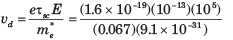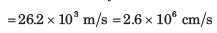Test: Semiconductor Physics - Question 16

A gallium arsenide semiconductor at T = 300 K is doped with impurity concentration Nd = 1016 cm-3 The mobility μn is 7500 cm2/ V - s  . For an applied field of 10 V/cm the drift current density is

Detailed Solution for Test: Semiconductor Physics - Question 16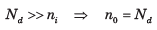J = eμn n0E = (1.6 x 10-19)(7500)(1016)(10) = 120 A/cm2

Test: Semiconductor Physics - Question 17

In a particular semiconductor the donor impurity concentration is Nd = 1014 cm-3. Assume the following parameters,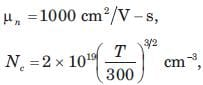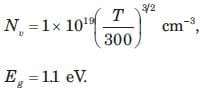An electric field of E = 10 V cm is applied. The electric current density at 300 K is

Detailed Solution for Test: Semiconductor Physics - Question 17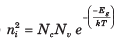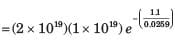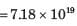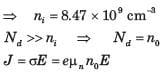=(1.6 x 10-19)(1000)(1014)(100) = 1.6 A/cm2

Test: Semiconductor Physics - Question 18

A semiconductor has following parameter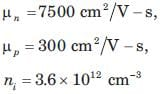Q. When conductivity is minimum, the hole concentration is

Detailed Solution for Test: Semiconductor Physics - Question 18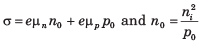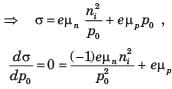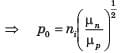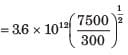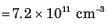Test: Semiconductor Physics - Question 19

A semiconductor has following parameter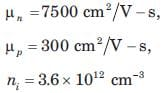Q. The minimum conductivity is

Detailed Solution for Test: Semiconductor Physics - Question 19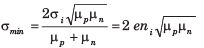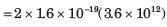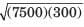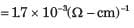Test: Semiconductor Physics - Question 20

A particular intrinsic semiconductor has a resistivity of 50 (Ω - cm) at T = 300 K and 5(Ω - cm) at T = 330 K. If change in mobility with temperature is neglected, the bandgap energy of the semiconductor is

Detailed Solution for Test: Semiconductor Physics - Question 20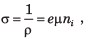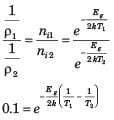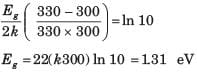## RRB JE for Electrical Engineering

2 videos|3 docs|77 tests
Information about Test: Semiconductor Physics Page
In this test you can find the Exam questions for Test: Semiconductor Physics solved & explained in the simplest way possible. Besides giving Questions and answers for Test: Semiconductor Physics, EduRev gives you an ample number of Online tests for practice

## RRB JE for Electrical Engineering

2 videos|3 docs|77 tests